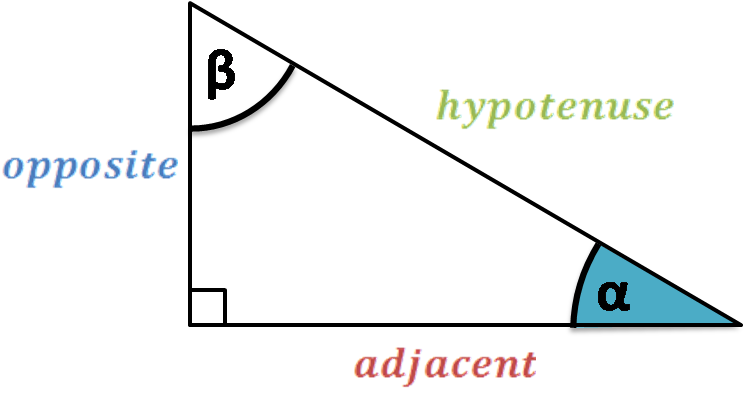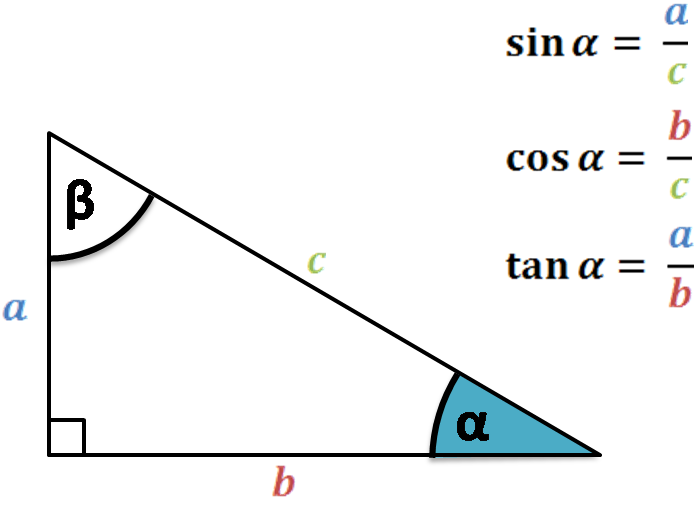# Trig Function Calculator

Created by Luis Hoyos
Reviewed by Davide Borchia
Last updated: May 19, 2023

This trig function calculator prints the value of the six trig functions given the angle in degrees or radians. In the article below, we explain:

• What are the 6 trig functions; and
• How to calculate those trig functions.

In the FAQ section, we present the value of the trig functions for some of the most common angles: the values of the six trig functions for 45 degrees and 270 degrees.

## The 6 trig functions.

#### Sine, cosine, and tangent

Sine, cosine, and tangent are the trigonometric functions of an angle. The most common way to define them is by using a right triangle and one of its angles (the angle of interest).

First, let's remember the names of the three sides of a right triangle:• Hypotenuse: The longest side, always opposite to the right angle;
• Opposite side: The side opposite to the angle of interest (α); and
• Adjacent side: The side of the triangle that is neither the hypotenuse nor the opposite side.

As you can note, the hypotenuse and adjacent side form the reference angle (α).

Then, the trigonometric functions are defined as:

sin(α) = opposite/hypotenuse

cos(α) = adjacent/hypotenuse

tan(α) = opposite/adjacent🙋 In summary, we use the right triangle to easily define the reference angle and calculate the trigonometric functions based on the lengths of the sides.

#### Cosecant, secant, and cotangent

Cosecant (csc), secant (sec), and cotangent (cot) are simply the reciprocals of the three previous functions:

• csc(α) = 1/sin(α) = hypotenuse/opposite

• sec(α) = 1/cos(α) = hypotenuse/adjacent

• cot(α) = 1/tan(α) = adjacent/opposite

## FAQ

### What are the values of the six trig functions for 45 degrees?

The values of the six trig functions for 45 degrees are:

• sin(45°) = √2/2 ≈ 0.70710678
• cos(45°) = √2/2 ≈ 0.70710678 (the same as sin(45°))
• tan(45°) = 1
• sec(45°) = √2 ≈ 1.41421356
• csc(45°) = √2 ≈ 1.41421356 (the same as sec(45°))
• cot(45°) = 1

### What are the values of the 6 trig functions for 270 degrees?

The values of the 6 trig functions for 270 degrees are:

• sin(270°) = -1
• cos(270°) = 0
• tan(270°) = undefined
• sec(270°) = 0
• csc(270°) = undefined
• cot(270°) = -1
Luis Hoyos
Angle
deg
People also viewed…

### Car heat

The hot car calculator shows how fast a car's interior heats up during a summer day.

### Circle skirt

Circle skirt calculator makes sewing circle skirts a breeze.

### Cube Calc: find v, a, d

This cube calc finds V (volume), a (area) and d (diagonal) of a cube.

### Heptagon

Find the area, perimeter, or length of sides for a heptagon using our heptagon calculator.